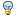Differences between revisions 4 and 5
 ⇤ ← Revision 4 as of 2017-12-01 18:10:37 → Size: 1491 Editor: KeithLofstrom Comment: ← Revision 5 as of 2017-12-01 18:11:14 → ⇥ Size: 1504 Editor: KeithLofstrom Comment: Deletions are marked like this. Additions are marked like this. Line 28: Line 28: $a = 9.81 \times gee = \omega^2 R = { \Large \left( { 2 \pi } \over T \right) }^2 R = 4 \pi^2 { \Large { R \over T^2 } } ~~~ T$ in seconds $a = 9.81 \times gee ~=~ \omega^2 R ~=~ { \Large \left( { 2 \pi } \over T \right) }^2 R ~=~ 4 \pi^2 { \Large { R \over T^2 } } ~~~ T$ in seconds Line 30: Line 30: $gee \times T^2 \approx 4 R ~~~~~ T = 60 / RPM$ $gee \times T^2 \approx 4 R ~~~~~ T ~=~ 60 / RPM$ Line 32: Line 32: $R = { \Large \left( 30 \over RPM \right)^2 } gee$ $R ~=~ { \Large \left( 30 \over RPM \right)^2 } gee$

# Gee Plus

## The Experiment

A 36 meter radius, rotating long-duration space habitat simulation on Earth, simulating 1.4 gees at 10 RPM. 1.4 gees is the vector sum of 1 gee horizontal and 1 gee vertical. Experimental subjects with BMI < 20, having a "gravitational BMI" < 28 but the same "metabolic BMI".

## Postulates

• 1 Humans evolved to run, hence may be optimized for > 1 gee

• see work by Joan Vernikos, NASA Ames (retired)
• zero gee causes rapid "aging"
• 2 The human vestibular system can adapt to high RPMS
• Experiments with rotating rooms show 6 RPM adaptation in 3 days, 10 RPM in 5 days
• Experiments with rotating tube beds suggest 30 RPMadaptation for head movements

• Athletes undergo much faster head rotations

## Goals

• Test the Vernikos theory: do healthy humans do better in > 1 gee environments?

• Learn about long term vestibular adaption, and the transition from rotation to non-rotation
• frequent transitions through the hub to 1 gee and 0 RPM
• Select astronauts for vestibular tolerance for rotating habitats in microgravity
• Make low BMI people into heros

### Math

a = 9.81 \times gee ~=~ \omega^2 R ~=~ { \Large \left( { 2 \pi } \over T \right) }^2 R ~=~ 4 \pi^2 { \Large { R \over T^2 } } ~~~ T in seconds

gee \times T^2 \approx 4 R ~~~~~ T ~=~ 60 / RPM

R ~=~ { \Large \left( 30 \over RPM \right)^2 } gee

GeePlus (last edited 2017-12-01 18:21:08 by KeithLofstrom)